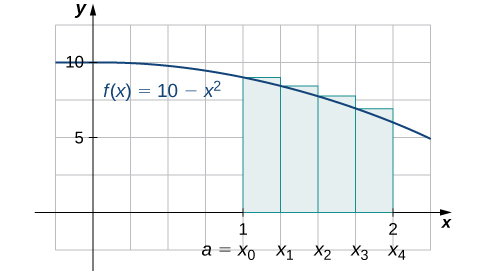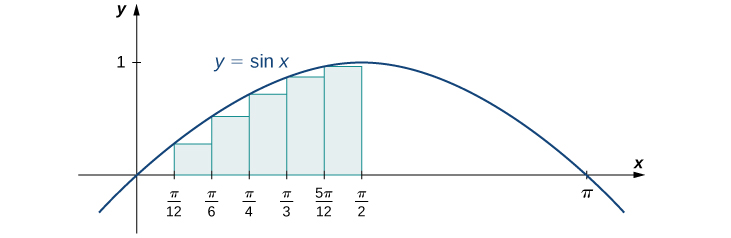# 5.1 Approximating areas  (Page 7/17)

 Page 7 / 17
1. Find an upper sum for $f\left(x\right)=10-{x}^{2}$ on $\left[1,2\right];$ let $n=4.$
2. Sketch the approximation.
1. $\text{Upper sum}=8.0313.$

2.## Finding lower and upper sums for $f\left(x\right)=\text{sin}\phantom{\rule{0.1em}{0ex}}x$

Find a lower sum for $f\left(x\right)=\text{sin}\phantom{\rule{0.1em}{0ex}}x$ over the interval $\left[a,b\right]=\left[0,\frac{\pi }{2}\right];$ let $n=6.$

Let’s first look at the graph in [link] to get a better idea of the area of interest.The graph of y = sin x is divided into six regions: Δ x = π / 2 6 = π 12 .

The intervals are $\left[0,\frac{\pi }{12}\right],\left[\frac{\pi }{12},\frac{\pi }{6}\right],\left[\frac{\pi }{6},\frac{\pi }{4}\right],\left[\frac{\pi }{4},\frac{\pi }{3}\right],\left[\frac{\pi }{3},\frac{5\pi }{12}\right],$ and $\left[\frac{5\pi }{12},\frac{\pi }{2}\right].$ Note that $f\left(x\right)=\text{sin}\phantom{\rule{0.1em}{0ex}}x$ is increasing on the interval $\left[0,\frac{\pi }{2}\right],$ so a left-endpoint approximation gives us the lower sum. A left-endpoint approximation is the Riemann sum $\sum _{i=0}^{5}\text{sin}\phantom{\rule{0.1em}{0ex}}{x}_{i}\left(\frac{\pi }{12}\right).$ We have

$\begin{array}{cc}A\hfill & \approx \text{sin}\left(0\right)\left(\frac{\pi }{12}\right)+\text{sin}\left(\frac{\pi }{12}\right)\left(\frac{\pi }{12}\right)+\text{sin}\left(\frac{\pi }{6}\right)\left(\frac{\pi }{12}\right)+\text{sin}\left(\frac{\pi }{4}\right)\left(\frac{\pi }{12}\right)+\text{sin}\left(\frac{\pi }{3}\right)\left(\frac{\pi }{12}\right)+\text{sin}\left(\frac{5\pi }{12}\right)\left(\frac{\pi }{12}\right)\hfill \\ & =0.863.\hfill \end{array}$

Using the function $f\left(x\right)=\text{sin}\phantom{\rule{0.1em}{0ex}}x$ over the interval $\left[0,\frac{\pi }{2}\right],$ find an upper sum; let $n=6.$

$A\approx 1.125$

## Key concepts

• The use of sigma (summation) notation of the form $\sum _{i=1}^{n}{a}_{i}$ is useful for expressing long sums of values in compact form.
• For a continuous function defined over an interval $\left[a,b\right],$ the process of dividing the interval into n equal parts, extending a rectangle to the graph of the function, calculating the areas of the series of rectangles, and then summing the areas yields an approximation of the area of that region.
• The width of each rectangle is $\text{Δ}x=\frac{b-a}{n}.$
• Riemann sums are expressions of the form $\sum _{i=1}^{n}f\left({x}_{i}^{*}\right)\text{Δ}x,$ and can be used to estimate the area under the curve $y=f\left(x\right).$ Left- and right-endpoint approximations are special kinds of Riemann sums where the values of $\left\{{x}_{i}^{*}\right\}$ are chosen to be the left or right endpoints of the subintervals, respectively.
• Riemann sums allow for much flexibility in choosing the set of points $\left\{{x}_{i}^{*}\right\}$ at which the function is evaluated, often with an eye to obtaining a lower sum or an upper sum.

## Key equations

• Properties of Sigma Notation
$\sum _{i=1}^{n}c=nc$
$\sum _{i=1}^{n}c{a}_{i}=c\sum _{i=1}^{n}{a}_{i}$
$\sum _{i=1}^{n}\left({a}_{i}+{b}_{i}\right)=\sum _{i=1}^{n}{a}_{i}+\sum _{i=1}^{n}{b}_{i}$
$\sum _{i=1}^{n}\left({a}_{i}-{b}_{i}\right)=\sum _{i=1}^{n}{a}_{i}-\sum _{i=1}^{n}{b}_{i}$
$\sum _{i=1}^{n}{a}_{i}=\sum _{i=1}^{m}{a}_{i}+\sum _{i=m+1}^{n}{a}_{i}$
• Sums and Powers of Integers
$\sum _{i=1}^{n}i=1+2+\text{⋯}+n=\frac{n\left(n+1\right)}{2}$
$\sum _{i=1}^{n}{i}^{2}={1}^{2}+{2}^{2}+\text{⋯}+{n}^{2}=\frac{n\left(n+1\right)\left(2n+1\right)}{6}$
$\sum _{i=0}^{n}{i}^{3}={1}^{3}+{2}^{3}+\text{⋯}+{n}^{3}=\frac{{n}^{2}{\left(n+1\right)}^{2}}{4}$
• Left-Endpoint Approximation
$A\approx {L}_{n}=f\left({x}_{0}\right)\text{Δ}x+f\left({x}_{1}\right)\text{Δ}x+\text{⋯}+f\left({x}_{n-1}\right)\text{Δ}x=\sum _{i=1}^{n}f\left({x}_{i-1}\right)\text{Δ}x$
• Right-Endpoint Approximation
$A\approx {R}_{n}=f\left({x}_{1}\right)\text{Δ}x+f\left({x}_{2}\right)\text{Δ}x+\text{⋯}+f\left({x}_{n}\right)\text{Δ}x=\sum _{i=1}^{n}f\left({x}_{i}\right)\text{Δ}x$

State whether the given sums are equal or unequal.

1. $\sum _{i=1}^{10}i$ and $\sum _{k=1}^{10}k$
2. $\sum _{i=1}^{10}i$ and $\sum _{i=6}^{15}\left(i-5\right)$
3. $\sum _{i=1}^{10}i\left(i-1\right)$ and $\sum _{j=0}^{9}\left(j+1\right)j$
4. $\sum _{i=1}^{10}i\left(i-1\right)$ and $\sum _{k=1}^{10}\left({k}^{2}-k\right)$

a. They are equal; both represent the sum of the first 10 whole numbers. b. They are equal; both represent the sum of the first 10 whole numbers. c. They are equal by substituting $j=i-1.$ d. They are equal; the first sum factors the terms of the second.

In the following exercises, use the rules for sums of powers of integers to compute the sums.

$\sum _{i=5}^{10}i$

$\sum _{i=5}^{10}{i}^{2}$

$385-30=355$

Suppose that $\sum _{i=1}^{100}{a}_{i}=15$ and $\sum _{i=1}^{100}{b}_{i}=-12.$ In the following exercises, compute the sums.

$\sum _{i=1}^{100}\left({a}_{i}+{b}_{i}\right)$

$\sum _{i=1}^{100}\left({a}_{i}-{b}_{i}\right)$

$15-\left(-12\right)=27$

$\sum _{i=1}^{100}\left(3{a}_{i}-4{b}_{i}\right)$

$\sum _{i=1}^{100}\left(5{a}_{i}+4{b}_{i}\right)$

$5\left(15\right)+4\left(-12\right)=27$

In the following exercises, use summation properties and formulas to rewrite and evaluate the sums.

$\sum _{k=1}^{20}100\left({k}^{2}-5k+1\right)$

$\sum _{j=1}^{50}\left({j}^{2}-2j\right)$

$\sum _{j=1}^{50}{j}^{2}-2\sum _{j=1}^{50}j=\frac{\left(50\right)\left(51\right)\left(101\right)}{6}-\frac{2\left(50\right)\left(51\right)}{2}=40,\text{​}375$

$\sum _{j=11}^{20}\left({j}^{2}-10j\right)$

$\sum _{k=1}^{25}\left[{\left(2k\right)}^{2}-100k\right]$

$4\sum _{k=1}^{25}{k}^{2}-100\sum _{k=1}^{25}k=\frac{4\left(25\right)\left(26\right)\left(51\right)}{9}-50\left(25\right)\left(26\right)=-10,\text{​}400$

f(x)=1/1+x^2 |=[-3,1]
apa itu?
fauzi
determine the area of the region enclosed by x²+y=1,2x-y+4=0
Hi
MP
Hi too
Vic
hello please anyone with calculus PDF should share
Which kind of pdf do you want bro?
Aftab
hi
Abdul
can I get calculus in pdf
Abdul
How to use it to slove fraction
Hello please can someone tell me the meaning of this group all about, yes I know is calculus group but yet nothing is showing up
Shodipo
You have downloaded the aplication Calculus Volume 1, tackling about lessons for (mostly) college freshmen, Calculus 1: Differential, and this group I think aims to let concerns and questions from students who want to clarify something about the subject. Well, this is what I guess so.
Jean
Im not in college but this will still help
nothing
how can we scatch a parabola graph
Ok
Endalkachew
how can I solve differentiation?
with the help of different formulas and Rules. we use formulas according to given condition or according to questions
CALCULUS
For example any questions...
CALCULUS
what is the procedures in solving number 1?
review of funtion role?
for the function f(x)={x^2-7x+104 x<=7 7x+55 x>7' does limx7 f(x) exist?
find dy÷dx (y^2+2 sec)^2=4(x+1)^2
Integral of e^x/(1+e^2x)tan^-1 (e^x)
why might we use the shell method instead of slicing
fg[[(45)]]²+45⅓x²=100
find the values of c such that the graph of f(x)=x^4+2x^3+cx^2+2x+2
anyone to explain some basic in calculus
I can
Debdoot
A conical container of radius 10 ft and height 30 ft is filled with water to a depth of 15 ft. How much work is required to pump all the water out through a hole in the top of the container if the unit weight of the water is 62.4 lb/ft^3?
hi am new here I really wants to know how the solve calculus
IBRAHIM
me too. I want to know calculation involved in calculus.
KatibaBy John GabrieliBy OpenStaxBy OpenStaxBy Saylor FoundationBy Brooke DelaneyBy Katherina jennife...By OpenStaxBy Kimberly NicholsBy Darlene PaliswatBy Richley Crapo